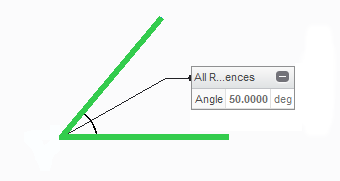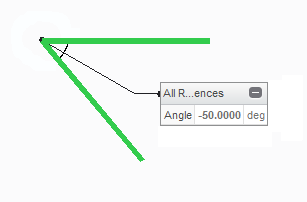Example: Angle Range
The angle range sets the range for updating an angle measurement in a saved analysis or feature if the model is redefined. If one reference entity moves to the other side of the second reference entity during redefinition, the angle measurement could change depending on the Range setting.
Start with an angle of 50º, measure the angle, and save the measurement as a feature.Move one reference curve to the other side of the second reference curve. If the angle range is set to +/-180, the resulting angle is -50º.If the angle range is set to 0-360, the resulting angle is 310º.